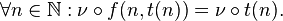# The most beautiful formulae in maths & science

I just though i'd make up a list of a few of the really beautiful formulas in mathematics.

One in my list is:

$$e^{i\pi} + 1 = 0$$

and definately, the Pythagoras theorem... as for physics.. the energy-mass equivalence is another beautiful formula:

$$E = mc^{2}$$

and.. the Bohr's formula for quantized angular momentum:

$$L = \frac{nh}{2\pi}$$

I also think that the Dirac equation is a beautiful result as it predicted the existence of a positron before it's actual discovery..

any others u can think of??

lisab
Staff Emeritus
Gold Member
You already have my favorite- Euler's.

There's a lot of ugly ones, too.

No object is so beautiful that, under certain conditions, it will not look ugly. - Oscar Wilde.

http://img401.imageshack.us/img401/2388/homeworkallforcesbh1.jpg [Broken]

Last edited by a moderator:
lisab
Staff Emeritus
Gold Member
No object is so beautiful that, under certain conditions, it will not look ugly. - Oscar Wilde.

http://img401.imageshack.us/img401/2388/homeworkallforcesbh1.jpg [Broken]
[/URL]

:rofl:

Last edited by a moderator:
George Jones
Staff Emeritus
Gold Member
No object is so beautiful that, under certain conditions, it will not look ugly. - Oscar Wilde.

http://img401.imageshack.us/img401/2388/homeworkallforcesbh1.jpg [Broken]
[/URL]

There's a sign mistake for the term a third of the way through the line that's a quarter of the way down.

Dark matter found!

Last edited by a moderator:
lisab
Staff Emeritus
Gold Member
"...sans gravity..."!

Well duh...wouldn't want to complicate it! :rofl:

A formula doesn't strike beautiful, an idea does though.

What book is that from? You've got me interested now.

Probably a joke, note the "Excercise #1.1.1.1.1.1.a"

robphy
Homework Helper
Gold Member
Kurdt
Staff Emeritus
Gold Member
The Schrödinger equation is pretty nice.

For sheer actual physical good looks, and usefulness I'd say the Laplacian looks quite nice.

$$\displaystyle \Delta = \frac{\partial^2 f}{\partial x^2} + \frac{\partial^2 f}{\partial y^2} + \frac{\partial^2 f}{\partial z^2}$$

Well, if I were a genius indian mathematician I'd say $$\sum_{n=1}^{\infty }n=-\frac{1}{12}$$

Maybe my eyes are just bad, but that isn't an equation, it is meaningless.

one of my favs.-Last edited by a moderator:
Pythagorean
Gold Member
For sheer actual physical good looks, and usefulness I'd say the Laplacian looks quite nice.

$$\displaystyle \Delta = \frac{\partial^2 f}{\partial x^2} + \frac{\partial^2 f}{\partial y^2} + \frac{\partial^2 f}{\partial z^2}$$

shouldn't it be turned around, squared, and acting on something on the left side?

This one also helped the US win WWII and also has been called one of the most important advances in science in the 20th centurty

N2 + 3H2---------> 2NH3 (Fe/K2O/Al2O3/250 atm/500 C)

I've always been partial to the http://en.wikipedia.org/wiki/Leibniz_formula_for_pi" [Broken]:

$$\huge \mathbf{\pi} = 4 \sum_{n=0}^\infty \frac{(-1)^n}{2n + 1}$$​

Last edited by a moderator:
Maybe my eyes are just bad, but that isn't an equation, it is meaningless.
:rofl:
They were telling the same to Fourier.

Probably one of the most important and beautiful mathematical object is the Riemann zeta function.
Since you like to copy equations from wikipedia, maybe you can check it out for real from time to time. It is instructive.

EDIT BTW, I was refering to Ramanujan who was maybe one of the most prolific for genuinely beautiful formulae

Last edited:
:rofl:
They were telling the same to Fourier.

Probably one of the most important and beautiful mathematical object is the Riemann zeta function.
Since you like to copy equations from wikipedia, maybe you can check it out for real from time to time. It is instructive.

EDITBTW, I was refering to Ramanujan who was maybe one of the most prolific for guenuinely beautiful formulae

No, I said it because what was posted did not contain any equal sign, and hence it is impossible for it to be an equation. At least the riemann zeta function has an equal sign.

Yeah, but I think gravenewworld is right, Ramanujan wouldn't write [post=1636702]what you posted there[/post], which currently shows an infinite summation that doesn't converge on a limit being equal to -1/12. And oblique name dropping is more of a vice than referencing Wikipedia, btw.

Last edited:
wait wait wait. I accidentally deleted what I was referring to. When I said "that isn't an equation, it is meaningless" I was referring to what jimmy posted. As far as I can see there is no equals sign in it anywhere.

Ahhhh, sorry for the confusion... Indeed, the "Standard model lagrangian (density) = " is missing in this, plus it is partly in french (sans gravity) so it really does not make sens :rofl:

Not much of a formula, but more of a process. However, its hard to deny that they are beautiful.

http://en.wikipedia.org/wiki/Fourier_transform" [Broken]

Last edited by a moderator:
1=0.999999

[/tex]